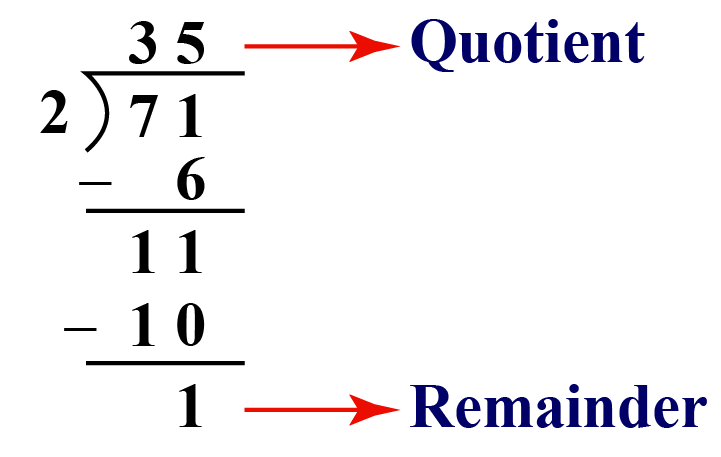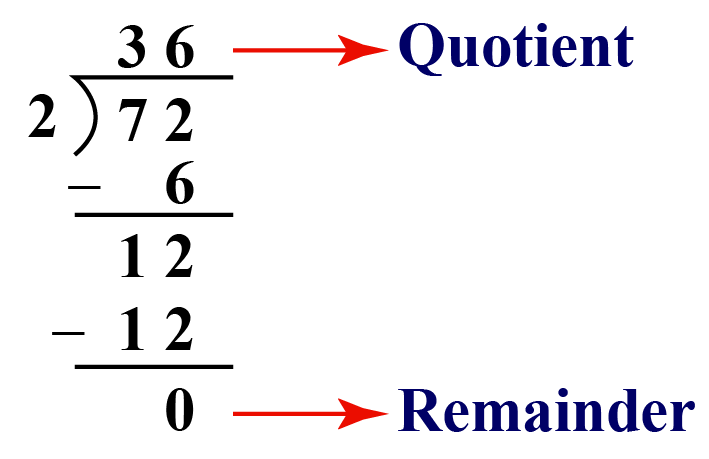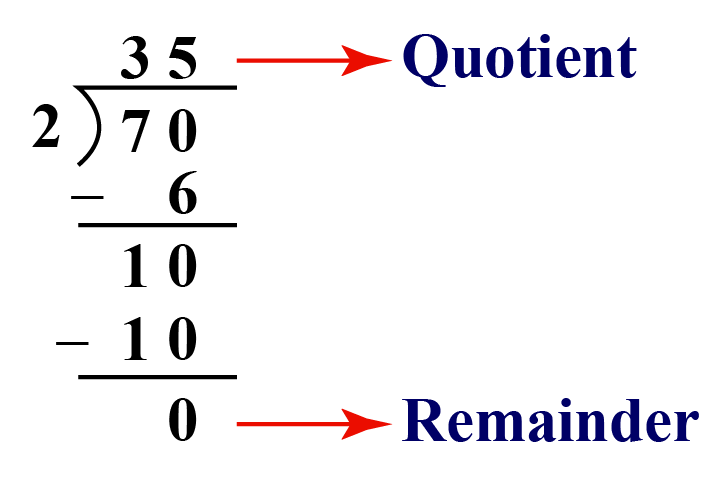# Is 71 A Prime Number?

Is 71 A Prime Number?
Go back to  'Prime Numbers'

In this mini-lesson, we will explore the world of prime numbers by finding the answers to the questions like is 71 a prime number, is 51 a prime number and is 97 a prime number while discovering the interesting facts around them.

Check out the interactive questions to know more about the lesson and try your hand at solving a few interesting practice questions at the end of the page.

Did you know, first written evidence of prime numbers was found in 1550 BC.

However, is it possible to identify a prime number just by looking at it? Basic rules are that except for 2, all prime numbers are odd. Also, a number will never be prime if the last digit is 5 because it will always be divisible by 5. That leaves you with 1, 3, 7, and 9

Now, let's help James who is struggling to identify if 71 is a prime number.## What Is A Prime Number?

Any whole number greater than 1, having only 2 factors, 1 and the number itself is known as a prime number.

For example; 23 is a prime number because it has only 2 factors 1 and the number itself, i.e. 23

## Is 71 A Prime Number?

Let us try dividing 71 by 2, we get,The remainder 1 shows that 71 is not entirely divisible by 2. This shows the number is odd.

If we similarly try dividing the number by 71 by 3, we get a remainder 2

When 71 is divided by 5, we get a remainder 1

It shows it isn't divisible other than 1 and 71

Hence, 71 can be factored as a product of 1 and 71 and be written as $$71 = 1 \times 71$$

## Solved Examples

 Example 1

Lucy has to list prime numbers between 70 and 100. Help her list them.

Solution

Lucy listed the following prime numbers between 70 and 100. They are:

71, 73, 79, 83, 89 and 97

She found there were 6 prime numbers between 70 to 100

 $$\therefore$$ Lucy listed 6 prime numbers between 70 and 100
 Example 2

Eric has to prove that 1 added or subtracted in 71, makes it a composite number. How will he prove it?

Solution

In addition to 1 to 71, Eric will get, 72. On subtraction of 1 from 71, he will get 70

He will check whether 72 is a prime number or not by dividing it by 2 in the following way:On division with 2, 72 leaves no remainder.

On division with 3, 72 leaves no remainder either.

But on division with 5, 72 leaves a remainder of 2, which shows it isn't divisible by 5

This shows it has factors other than 1 and 72

Factors of 72 are, 1, 2, 3, 4, 6, 8, 9, 12, 18, 24, 36 and 72

It has factors other than 1 and 72, which makes it a composite number.

He will check whether 70 is a prime number or not by dividing it by 2 in the following way:On division with 2, 70 leaves no remainder.

On division with 3, 70 leaves a remainder 1, which shows that 70 isn't divisible by 3

But on division with 5, 70 leaves no remainder.

This shows it has factors other than 1 and 70

Factors of 70 are, 1, 2, 5, 7, 10, 14, 35 and 70

It has factors other than 1 and 70, which makes it a composite number.

Hence 1 added or subtracted in 71, makes it a composite number.

 Hence Proved

## Interactive Questions

Here are a few activities for you to practice.Challenging Questions
• Leslie has to find prime numbers from 1 to 100 whose sum of digits is also a prime number.
• How will Ash check is 51 a prime number or not?

## Let's Summarize

The mini-lesson targeted the fascinating concept of prime numbers. The math journey around prime numbers starts with what a student already knows, and goes on to creatively crafting a fresh concept in the young minds. Done in a way that not only it is relatable and easy to grasp, but also will stay with them forever. Here lies the magic with Cuemath.

We hope you enjoyed learning about is 71 a prime number with the practice questions. Now you will be able to easily solve problems on prime numbers, is 51 a prime number and is 97 a prime number.

At Cuemath, our team of math experts is dedicated to making learning fun for our favorite readers, the students!

Through an interactive and engaging learning-teaching-learning approach, the teachers explore all angles of a topic.

Be it worksheets, online classes, doubt sessions, or any other form of relation, it’s the logical thinking and smart learning approach that we, at Cuemath, believe in.

## 1. What are co-prime numbers?

It is a set of any two numbers which have only 1 as their common factor. For example: 2 and 3 are co-prime numbers.

## 2. What is the prime factorization of 71?

The prime factorization of 71 is the product of prime factors of 71 which result in 71. The prime factorization of 71 is 1 and 71

## 3. Is 71 a prime number? Yes or No?

Yes, 71 is a prime number as it has only two factors: 1 and 71

More Important Topics
Numbers
Algebra
Geometry
Measurement
Money
Data
Trigonometry
Calculus